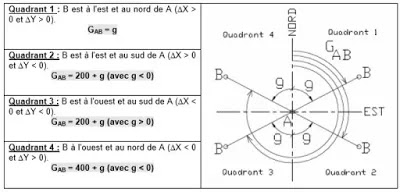How to calculate the coordinates of a vector ₪ 2.1 Calculation of a bearing from Cartesian coordinates

# How to calculate the coordinates of a vector ₪ 2.1 Calculation of a bearing from Cartesian coordinates

Consider the coordinates of two points (EANA) and B (EBNB BB)

The following relationship is used to calculate GAB :Exercise n°1 :
Calculate from the formula the bearing of the following direction AB with:
(10 ; 50) and B (60 10) the lines SA whose lenght Dp the operator has measured. It reads the corresponding angle V, it measures Dp and deduces that :